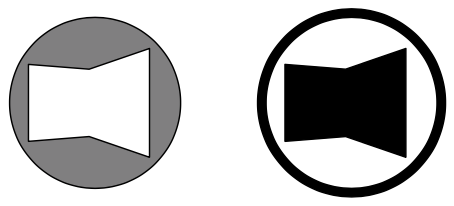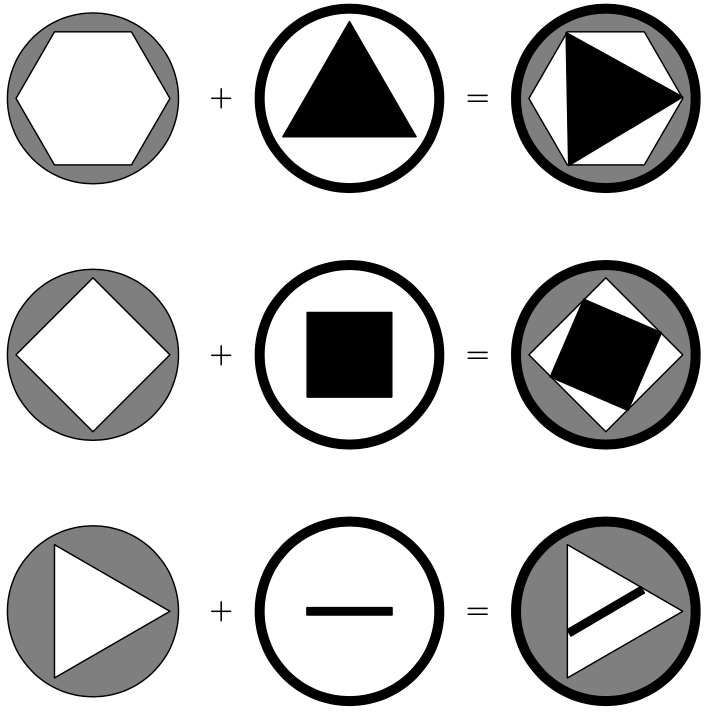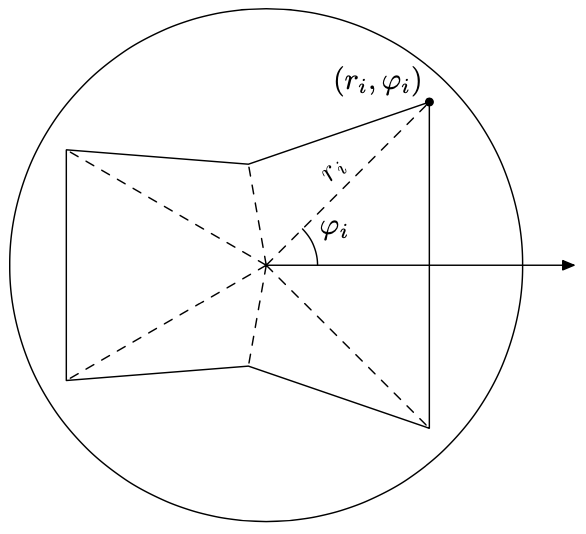시간 제한 메모리 제한 제출 정답 맞은 사람 정답 비율
3 초 256 MB 0 0 0 0.000%

## 문제

Tommy is a wheel thief. His job was formerly as easy as pie: you lift a car, turn off wheel bolts, take the wheel and run away. But now everybody uses "anti-theft" bolts.

Anti-theft bolt is designed in such a way that it cannot be turned off with a usual wrench. Its head is a cylinder with a hole. To turn the anti-theft bolt off you need a right wrench. The wrench has a ring with a lug that exactly matches the shape of the bolt head.Of course Tommy cannot get wrenches for all possible anti-theft bolts. But sometimes it is possible to turn off the bolt with the wrench that does not match it exactly.

More formally, the wrench can turn off the bolt if and only if two following conditions are satisfied:

• the ring of the wrench can be joined with the cylinder of the bolt head in such a way that the lug of the wrench is inside the hole of the bolt head;
• the wrench cannot make a full turn when the bolt is fixed.

For example:Situations where the bolt can be turned off with improper wrench.

Due to technical reasons, the shape of both --- hole of the bolt head and lug of the wrench, are always a star-shaped polygons with theirs centers in the center of the bolt or wrench. So if it is described in polar coordinate system as a sequence of pairs ($r_i, \varphi_i$) then $\varphi_{i + 1} < \varphi_i$ and $\varphi_{i + 1} - \varphi_i < 180^\circ$.Help Tommy do find out if it is possible to turn off the bolt with the wrenches he has.

## 입력

The first line of input file contains two integer numbers $n$ and $r$ --- the number of wrenches and the radii of the bolt head and the wrenches' rings ($1\le n\le 10$, $1\le R\le 1000$).

The following lines describe the bolt head. Description consists of an integer number $m$ --- number of vertices ($3\le m\le 100$) and $m$ pairs of integer numbers ($r_i, \varphi_i$) ($1\le r_i < R$; $0^\circ \le \varphi_i < 360^\circ$; $\varphi_i < \varphi_{i+1}$; $\varphi_{i+1} - \varphi_i < 180^\circ$; $\varphi_{m} - \varphi_1 > 180^\circ$).

The rest lines describe the wrenches in the same format.

## 출력

The first line of the output file must contain the number of wrenches that can be used to turn off the bolt. The following lines must contain wrench numbers in increasing order.

## 예제 입력 1

3 10
4
9 0
9 90
9 180
9 270
4
8 45
8 135
8 225
8 315
4
6 45
6 135
6 225
6 315
3
7 0
7 90
6 225


## 예제 출력 1

2
1 3